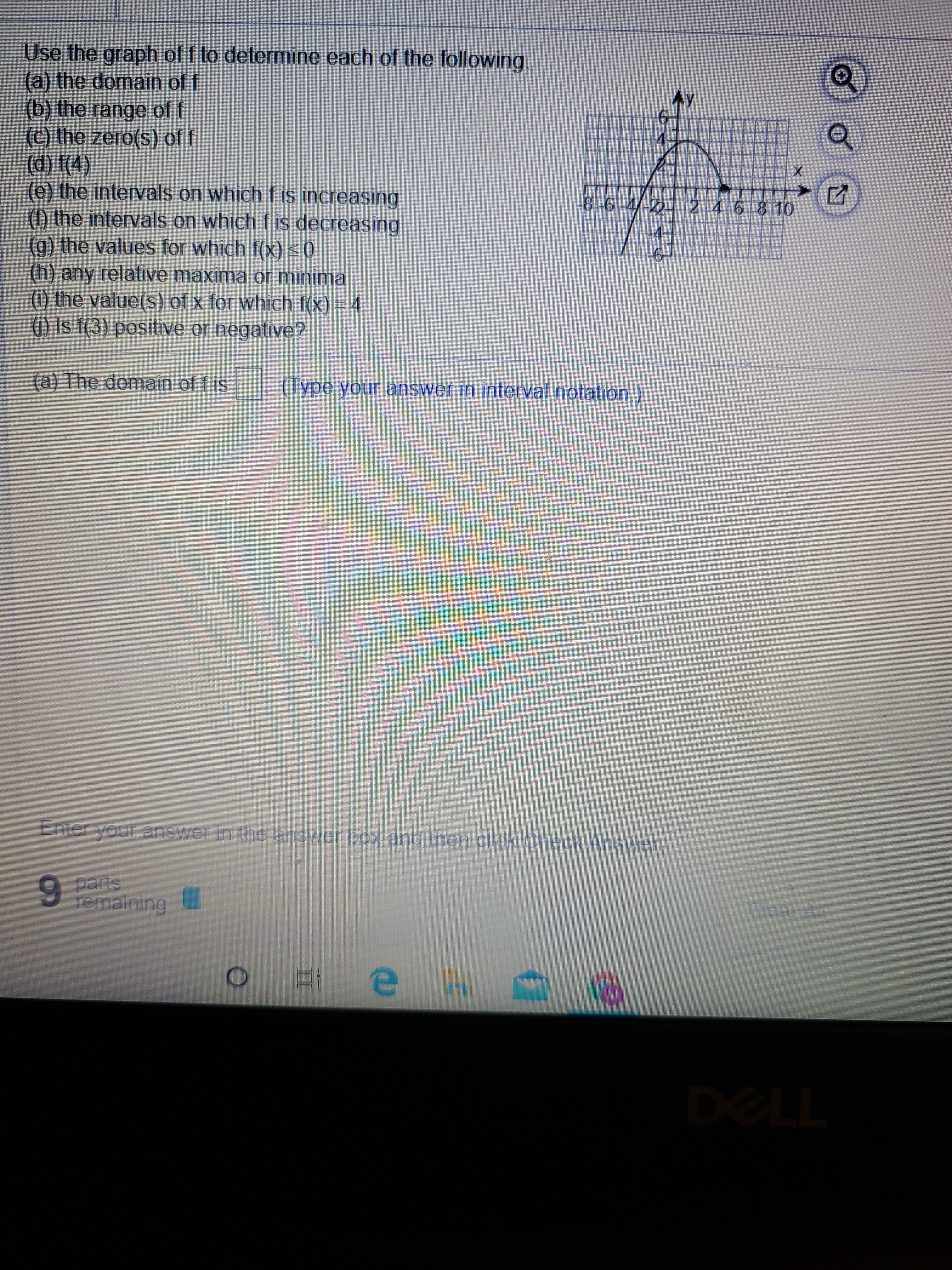# Use the graph of f to determine each of the following. (a) the domain of f (b) the range of f (c) the zero(s) of f (d) f(4) (e) the intervals on which f is increasing (f) the intervals on which f is decreasing

Question

I need help finding a through f, I know only 4 is allowed.  If someone can help me find a, b and c please .help_outlineImage TranscriptioncloseUse the graph of f to determine each of the following. (a) the domain of f (b) the range of f (c) the zero(s) of f (d) f(4) (e) the intervals on which f is increasing (f) the intervals on which f is decreasing fullscreen

### Want to see this answer and more?

Experts are waiting 24/7 to provide step-by-step solutions in as fast as 30 minutes!*

*Response times may vary by subject and question complexity. Median response time is 34 minutes for paid subscribers and may be longer for promotional offers.
Tagged in
Math
Calculus

### Functions